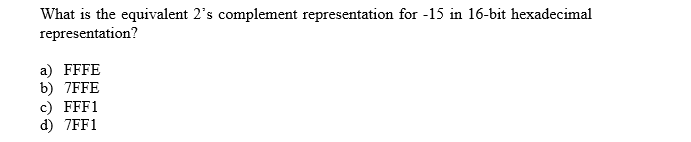What is the equivalent 2's complement representation for -15 in 16-bit hexadecimalrepresentation?a FFFEb) 7FFEc) FFF1d) 7FF1

Questionhelp_outlineImage TranscriptioncloseWhat is the equivalent 2's complement representation for -15 in 16-bit hexadecimal representation? a FFFE b) 7FFE c) FFF1 d) 7FF1 fullscreen
Step 1

For representing –15 in 2’s complement notation following steps should be followed:

1. Find the binary equivalent of 15.
2. Represent the equivalent binary representation using 16 bits.
3. Use the most significant bit for sign representation.
4. Get 2’s complement of the above binary representation.
5. Convert the obtained 2’s complement representation in hexadecimal form.
Step 2

Now,

The number 15 in binary form is represented as 1111. In 16-bit representation the number 15 will be represented as 0000 0000 0000 1111.

Now make the most significant bit of above decimal number as 1 since the number is negative. Therefore, –15 in 16-bit decimal representation will be represented as 1000 0000 0000 1111.

Step 3

Now to find the 2’s complement of the 16-bit representation of above number first find the 1’s complement and then add 1 to the result to obtain 2’s complement.

Now, 1’s complemen...

Want to see the full answer?

See Solution

Want to see this answer and more?

Our solutions are written by experts, many with advanced degrees, and available 24/7

See Solution
Tagged in

Other